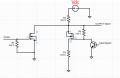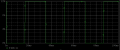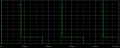# Using FET for pass PWM signal form Source to Drain

#### hyyee

Joined Jul 4, 2023
5
Hello !
I need some help for circuit.
I designed circuit such below, but that is not general.
I'm curious about role of R4 resistor in this circuit.---------------------------------------------------------------------------
The operation of circuit :
** R1 is pull-down resistor
case 1
@ PWM = high -> N ch mosfet : on
@ Input signal = High -> NPN Transistor ON -> P ch mosfet : on
=> OUTPUT = Ground (by N ch mosfet)

case 2
@ PWM = Low -> N ch mosfet : off
@ Input signal = High -> NPN Transistor ON -> P ch mosfet : on
=> OUTPUT = Vdc * {R3/(R2+R3)}

case 3
@ PWM = high -> N ch mosfet : on
@ Input signal = Low -> NPN Transistor off -> P ch mosfet : off
=> OUTPUT = Ground (by N ch mosfet)

case 4
@ PWM = Low -> N ch mosfet : off
@ Input signal = Low -> NPN Transistor off -> P ch mosfet : off
=> OUTPUT = Vdc
---------------------------------------------------------------------------
When I simulate the circuit in the image, it works as intended.However, if i remove the R4 resistor, case3/4 behaves strangely.And let me know if there's anything else I should consider in this circuit.#### ericgibbs

Joined Jan 29, 2010
18,226
Hi hyyee,
Welcome to AAC.

Is this a homework or college assignment.?

Moderation.

#### Veracohr

Joined Jan 3, 2011
771
R4 is connected between two points. Consider the significance of those two points.

#### crutschow

Joined Mar 14, 2008
33,355
A MOSFET gate must never be left floating as it's voltage is then undefined.

#### Irving

Joined Jan 30, 2016
3,548
Your circuit works, but not as you describe or intend.

Why do you need the NPN transistor AND the P-channel MOSFET to short the output to ground?

If R4 is null ohm = 0 ohm, then the P-Channel MOSFET is doing nothing useful.

If R2 is 0 ohm then you are shorting out your power supply, expect much magic smoke in the real world...

The simplicity of the simulation is lulling you into a false sense of security....

#### hyyee

Joined Jul 4, 2023
5
Hi hyyee,
Welcome to AAC.

Is this a homework or college assignment.?

Moderation.
I am HW engineer.
This is my seminar topic.#### hyyee

Joined Jul 4, 2023
5
R4 is connected between two points. Consider the significance of those two points.
Thank you for your response. What I'm wondering is why it takes a peak (12V) and then drops to 2V.

#### hyyee

Joined Jul 4, 2023
5
A MOSFET gate must never be left floating as it's voltage is then undefined.
Thank you for your response. What I'm wondering is why it's taking a peak (12V) and then dropping to 2V, can I understand it as a strange behavior caused by the gate being in floating state?

#### hyyee

Joined Jul 4, 2023
5
Your circuit works, but not as you describe or intend.

Why do you need the NPN transistor AND the P-channel MOSFET to short the output to ground?

If R4 is null ohm = 0 ohm, then the P-Channel MOSFET is doing nothing useful.

If R2 is 0 ohm then you are shorting out your power supply, expect much magic smoke in the real world...

The simplicity of the simulation is lulling you into a false sense of security....
The resistors are not 0R, they are undetermined values. Sorry for the confusion.